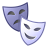PSoC™ 4Level 3Level 3

# Amux resistance

Hello,

I'm using the PSOC 4200M.  I designed a circuit using an opamp in non-inverting mode.  The Gain resistors are off chip.  I noticed that as I increase the resistors past a gain of one, the gain appears to increase in a non-linear fashion.  I'm using large value resistors to keep the current low.  I am also using an Analog mux to switch in the feedback resistors.

My guess is the internal resistance to the AMUX is a function of current. Here are the resistor values I'm using:

Rin (negative terminal) = 1 M Ohm

Feedback resistors switched in (one at a time) =  1 M ohm,  2.49 M ohms, 7.2 Mohms

These values equate to gains of 2, 3.49 and 8.2.  However, the actual gains calculated from measuring the input and output voltages of the opamp are 2, 4.38 and 11.59 respectively.

Is there a function that describes this behavior?

thanks

Shawn

1 SolutionLevel 9Level 9

# Re: Amux resistance